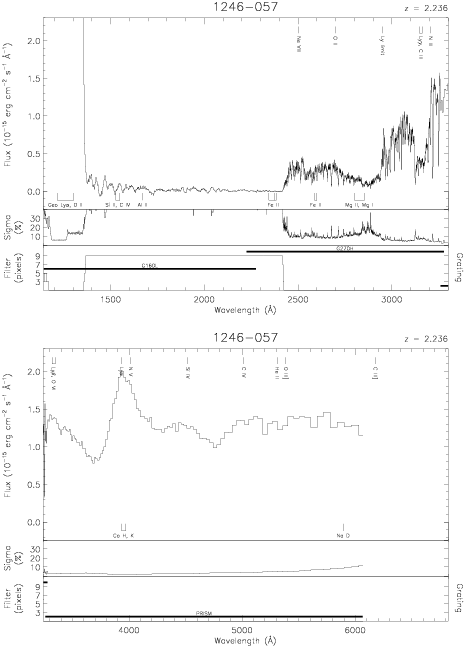Download dataset
TELESCOP= 'HST     '
INSTRUME= 'FOS     '
EQUINOX =               2000.0
DATE-OBS= '1992-11-26T05:42:36'
RA_PNT  =       192.3076833333
DEC_PNT =        -5.9887055556
PA_PNT  =       193.6512065543
OBJECT  = '1246-057'
ROOTNM01= 'Y1080102T'
DETECT01= 'BLUE    '
APERID01= 'A-1     '
FGWAID01= 'L15     '
ROOTNM02= 'Y1080103T'
DETECT02= 'BLUE    '
APERID02= 'A-1     '
FGWAID02= 'PRI     '
ROOTNM03= 'Y1HQ0104T'
GRNDMD03= 'SPECTROSCOPY'
DETECT03= 'AMBER   '
APERID03= 'B-3     '
FGWAID03= 'H27     '
ROOTNM04= 'Y1HQ0105T'
GRNDMD04= 'SPECTROSCOPY'
DETECT04= 'AMBER   '
APERID04= 'B-3     '
FGWAID04= 'H27     '
ROOTNM05= 'Y1HQ0106T'
GRNDMD05= 'SPECTROSCOPY'
DETECT05= 'AMBER   '
APERID05= 'B-3     '
FGWAID05= 'H27     '
ROOTNM06= 'Y1HQ0107T'
GRNDMD06= 'SPECTROSCOPY'
DETECT06= 'AMBER   '
APERID06= 'B-3     '
FGWAID06= 'H27     '